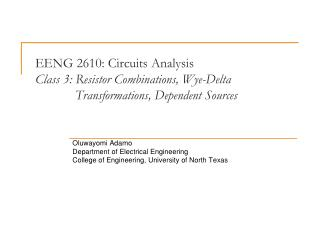DownloadDownload PresentationOluwayomi Adamo Department of Electrical Engineering

Oluwayomi Adamo Department of Electrical Engineering

Download PresentationOluwayomi Adamo Department of Electrical Engineering

- - - - - - - - - - - - - - - - - - - - - - - - - - - E N D - - - - - - - - - - - - - - - - - - - - - - - - - - -
Presentation Transcript

1. EENG 2610: Circuits AnalysisClass 3: Resistor Combinations, Wye-Delta Transformations, Dependent Sources Oluwayomi Adamo Department of Electrical Engineering College of Engineering, University of North Texas

2. Series and Parallel Resistor Combinations

3. Simplifying Resistor Combinations • To determine equivalent resistance at a pair of terminals of a network • Begin at the end of the network opposite the terminals • Repeat the following two steps as needed to reduce the network to a single resistor at the pair of terminals • Combine resistors in series • Combine resistors in parallel

4. Example 2.20: Determine resistance RAB

5. Resistor Specifications • Resistor Value • Standard resistor values are usually fixed, so to achieve a specific value, we need to combine standard value resistors in a certain configuration. (see Table 2.1 on page 45) • Tolerance • Typically, 5% and 10%, which specifies possible minimum and maximum resistance values • Power Rating • Specifies the maximum power that can be dissipated by the resistor. Typically, ¼ W, ½ W, 1 W, 2 W, …

6. Example 2.22: Find the range for both current and power dissipation in the resistor if R has a tolerance of 10%.

7. Analyzing Circuits with Single Source and Series-Parallel Combination of Resistors • Step 1 • Systematically reduce the resistive network so that the resistance seen by the source is represented by a single resistor • Step 2 • Determine the source current for a voltage source or the source voltage if a current source is present • Step 3 • Expand the network, retracing the simplification steps, and apply Ohm’s law, KVL, KCL, voltage division, and current division.

8. Wye-Delta Transformation Can you simplify it? Equivalent Transform For two networks to be equivalent at each corresponding pair of terminals, it is necessary that the resistance at the corresponding terminals be equal.

9. Circuits with Dependent Sources • Controlled sources are used to model many important physical devices • Problem Solving Strategy • When writing KVL and/or KCL equations for the network, treat the dependent sources as though it were an independent source. • Write the equation that specifies the relationship of the dependent source to the controlling parameter. • Solve the equations for the unknowns. Be sure that the number of linearly independent equations matches the number of unknowns. • Will see a lot of examples a little later.